# How to calculate IRR

Published: 25 October 2022

## how-to-calculate-IRR-banner.jpg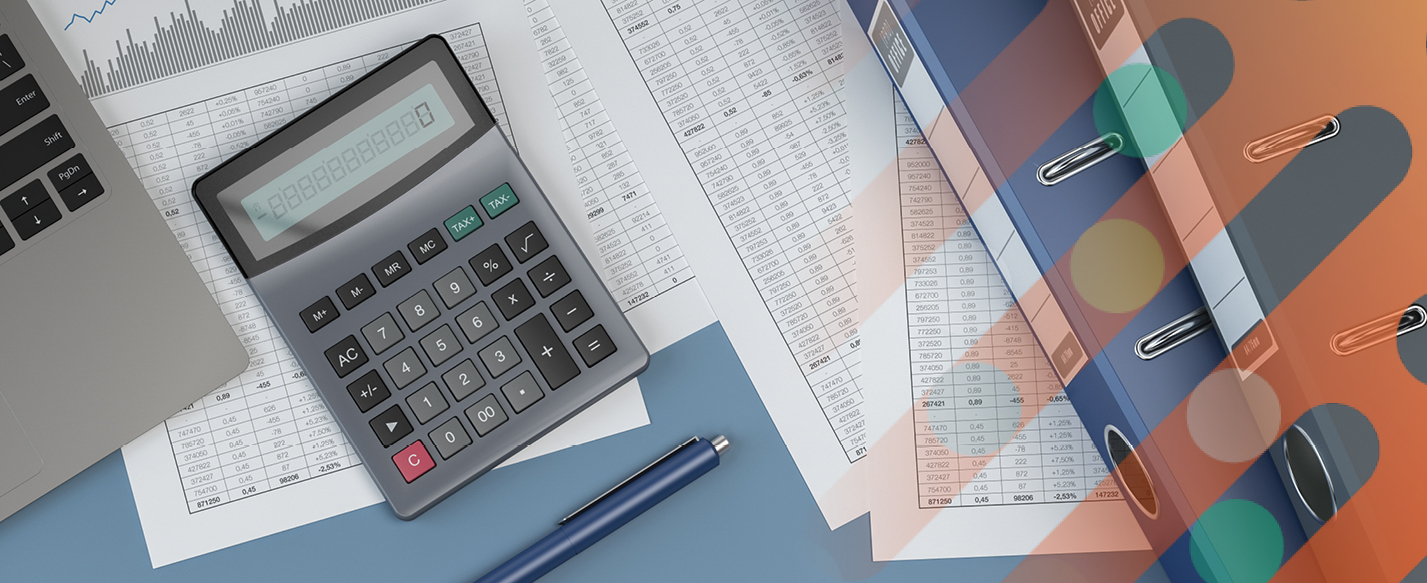IRR means Internal Rate of Return. In this article we focus on calculating IRR for investment proposals. However, the identical calculations apply for borrowings as well. When investing – all other things being equal – we prefer higher IRRs, meaning better rates of investment return. For borrowing, though – all other things, again, being equal – we prefer lower IRRs, meaning cheaper borrowing.

## Single cash flow at maturity

Let’s say Proposal A needs us to invest £100m today, to get back £121m in exactly 2 years time. What is the IRR per year of Proposal A?

Is there a formula for IRR in this case? Yes. The IRR formula we need is:

IRR = (End amount / Starting amount)(1/n) - 1
End amount = £121m
Starting amount = £100m
n = 2 years delay before final cash flow
IRR = (121/100)(1/2) – 1
= 1.21(1/2) – 1
= 1.10 – 1
= 0.10 = 10%

## Can we prove our answer of 10% IRR?

We can, indeed. IRR is defined as the cost of capital (r) that, when applied to discount all of the expected cash outflows and inflows in a proposal, results in a net present value (NPV) of zero.

From our calculation above, the IRR for Proposal A is 10%. Using this figure as our cost of capital (r) to discount Proposal A’s expected future cash flows, let’s see what the net present value is.

For each undiscounted future cash flow, the Discount Factor (DF) to apply to discount it to its present value is given by:

DF = 1/(1+r)n
Where:
r = cost of capital per period = 0.10
n = number of periods’ delay before cash flow

## Proof: net present value of Proposal A = £0m

Time (n years) Cash flow (out)/in £m x DF (=1/(1+r)n) = Present value £m
0 (100) 1/1.100 = 1.0000 (100)
2 121 1/1.102 = 0.8264 100
Total   NPV = 0

The net present value is zero, as expected. Consistent with our IRR formula.

### What if we could get our inflow sooner?

Now let’s say an improved alternative Proposal B similarly needs us to invest £100m today, to get back the same end cash amount of £121m. But under Proposal B we’ll get back the £121m in just one year’s time (rather than in 2 years as for Proposal A). What will be the effect of this timing change on the IRR?

### What is the IRR for Proposal B?

Again, for a single End value, after n = 1 period, the IRR is:

IRR = (End amount / Starting amount)(1/n) - 1
= (121/100)(1/1) – 1
= 1.21(1) – 1
= 1.21 – 1
= 0.21 = 21%

This is an improvement for us as the investor, compared with the 10% IRR for Proposal A.

### Proof: NPV of Proposal B = £0m

Time (n years) Cash flow (out)/in £m x DF (=1/(1+r)n) = Present value £m
0 (100) 1/1.210 = 1.0000 (100)
1 121 1/1.211 = 0.8264 100
Total   NPV = 0

Again, the NPV, using the IRR to discount with, is zero, as expected. So Proposal B does indeed have a superior IRR for us as the investor.

## Does that make sense?

Let’s set out each of Proposals A and B in a chart and compare them.

## Proposal-A-cash-returned-after-2-years-10%-irr.JPG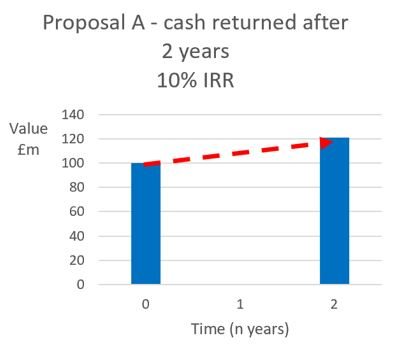Under Proposal A, we earn a surplus of £121m - £100m = £21m, over a 2-year period. The inferior rate of return of 10% IRR is indicated by the shallower slope of the broken red arrow. Our invested capital is locked in for the whole 2 years.

## Proposal-B-cash-returned-1-year-sooner-21%-irr.JPG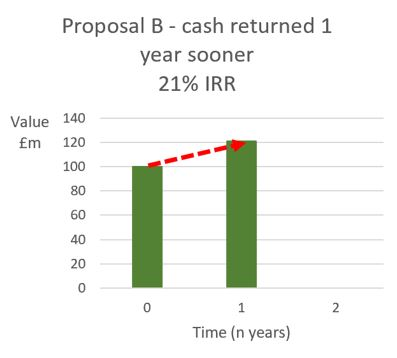On the other hand, with Proposal B, we earn the same amount of surplus, in cash terms, of £121m - £100m = £21m. Now over a single year. The superior rate of return of 21% IRR is shown by the steeper slope of the red arrow. We get our capital back a whole year sooner, and we can deploy our returned capital into other beneficial activities. This advantage for the investor is expressed in the higher IRR. That makes sense.

## Can IRR deal with multiple cash flows?

Yes, it can. But there isn’t any simple general formula that we can apply with a standard calculator. However, we can always work out IRR using a financial calculator or – preferably – a well-designed spreadsheet. An important advantage of well-designed spreadsheets is transparency and flexibility. In Excel, the formula for an IRR is = IRR( ).

For example, calculating the IRR for Proposal A in Excel:

## excel-sheet-1.JPG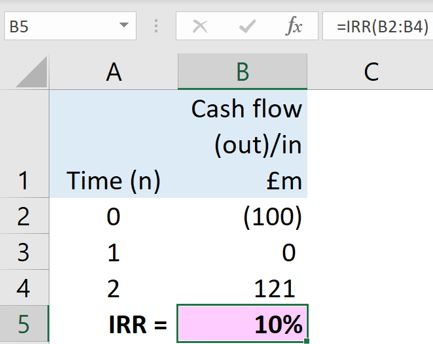The Excel formula we need in Cell B5 is =IRR(B2:B4).

If our spreadsheet is well designed, it’s easy to copy a worksheet and modify it to deal with alternative proposals, for example Proposal B:

## excel-sheet-2.JPG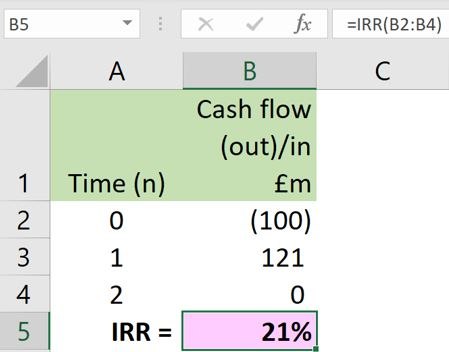## Can we deal with any pattern of cash flows?

Yes. Let’s extend our time frame to 4 years, and calculate the IRR for a 4-year bond with the following cash flows:

Current market value Time 0 = €(100,000) outflow.

Annual interest coupon Times 1 to 4 = €5,000 inflows.

Redemption value at Time 4 = €100,000 inflow.

Total receivable by investor at Time 4 = interest €5,000 + €100,000 redemption value = €105,000 inflow.

The current market value of €100,000 is an OUTFLOW for the investor.

Now putting all these cash flows into an extended copy of our spreadsheet:

## excel-sheet-3.JPG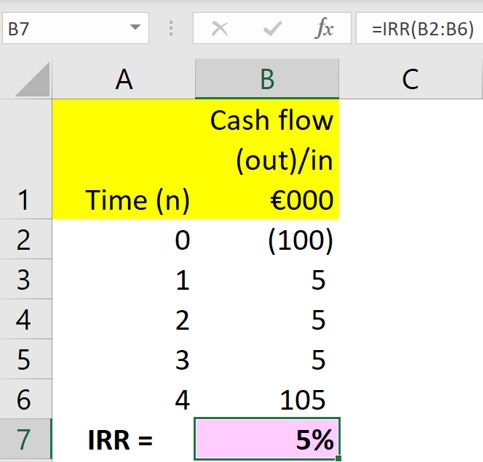Our spreadsheet model is saying the IRR for the investor is 5%. This figure is also known as the yield on the bond. This model can also deal with any other pattern of cash flows that we want to analyse.

## Can we prove the IRR of 5% for the bond?

Yes. As before, if we use a cost of capital (r) equal to the IRR to discount all the cash flows, the net present value (NPV) will be zero if our IRR is right.

## excel-sheet-4.JPG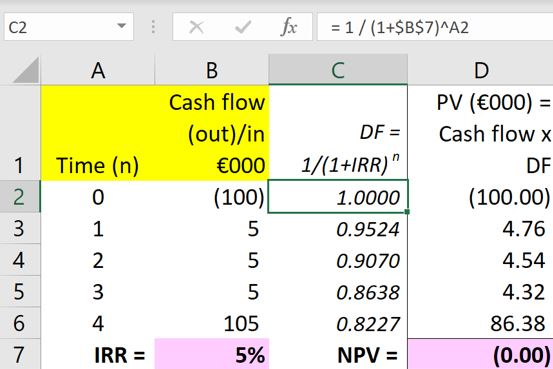That’s consistent. Again, our net present value is zero, as expected.

The Excel formula we need in Cell C2 to work out the first discount factor (DF) is:

= 1 / (1+\$B\$7)^A2

Then, copying this formula down the column of discount factors, the reference to the IRR cell B7 is anchored (fixed) so that we always use the same IRR = (r) to discount with, but the reference to the Time (n) column changes nicely in turn as we go down the column of discount factors:

## excel-sheet-5.JPG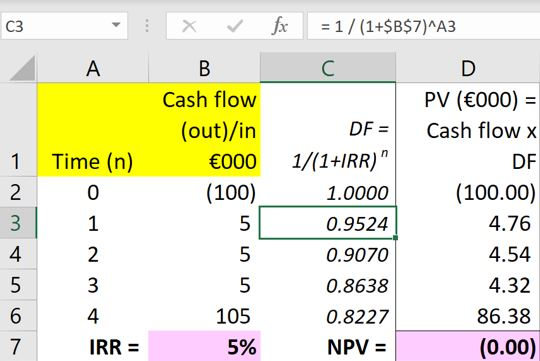The discount factor calculation in Cell C3 picks up the timing (n) from Cell A3, and so on.

## If the price of the bond were to fall, would its IRR go up?

Let’s see. The way to test that is to reduce the value of the bond in the model. For example, let’s assume the bond value falls to €99,000. Updating the inputs in our model:

## excel-sheet-6.JPGFollowing a fall in the market value of the bond to €99,000, the yield (IRR) has indeed gone up as expected, from 5% to 5.28%.

## Can we work out IRRs manually?

Yes, we can. The method for calculating IRRs without using Excel involves estimating an IRR to start with, calculating the resulting net present value manually, and then refining our next estimate - depending on the result of the first one.

For example, we might make a first estimate of 5% for the IRR of the bond trading at €99,000 in our example above. If we did that, we would use r = 0.05 to calculate a net present value of +€1,000 manually as follows:

Time (n years) Cash flow (out)/in €000 x DF (=1/(1+r)n) = Present value €000
0 (99) 1/1.050 = 1.0000 (99.00)
1 5 1/1.051 = 0.9524 4.76
2 5 1/1.052 = 0.9070 4.54
3 5 1/1.053 = 0.8638 4.32
4 105 1/1.054 = 0.8227 86.38
IRR estimate = 5% = 0.05 NPV = +1.00

The NPV is positive €1,000. So, our estimate of the IRR is too low. Now we would make another – higher – estimate and repeat the process.

## Iteration

Making successively better estimates in this way is known as “iteration”. This means we’re using the results of our last calculation as inputs for our next calculation. So long as we repeat the iteration process often enough, it will produce a fully accurate answer. We can do this for any pattern of cash flows.

## Interpolation

To speed up our repeated estimation and iteration process, we can also use the results of both of our first two estimates, to calculate our third estimate, and so on.

For example, let’s say our second estimated IRR produced a negative NPV of exactly €(1,000), following our initial estimate of 5% that produced a positive NPV of +€1,000. Our third estimate would then be exactly mid-way between our first estimate of 5% and our second estimated IRR. This is known as “straight line interpolation”.

## Invest in yourself for superior returns

___________________

Author: Doug Williamson, FCT

___________________

## Are you ready to start learning?

Choose from:

eLearning courses: 45-90 minutes to complete, available online 24/7.

training courses: live skills-based training sessions.

Treasury and Cash Management qualifications: internationally recognised courses from entry to master level. All our courses will provide you with valuable knowledge as well as easy-to-share digital credentials to demonstrate your learning achievements.

## More resources

If you found this article helpful, you might also be interested in our learning resource how to evaluate investments with IRR by Doug Williamson.